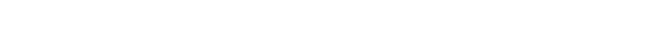Ewa Damek
Research
Dydaktyka

Research Interests

1. Random branching and affine structures

1. Affine stochastic recursions, Lipschitz iterations, global and local regularity of stationary measures.
2. Perpetuities, their path properties and related large deviations.
3. Multivariate stochastic models with power law tails.
4. Univariate and multivariate smoothing transforms.
5. Branching processes in random environment.

2. Harmonic functions on solvable NA groups

1. Theory of Poisson-Furstenberg boundaries for Hörmander type left-invariant differential operators on NA groups.
2. Analysis on harmonic manifolds.
3. Sharp pointwise estimates for Green functions and Poisson kernels for left-invariant differential operators on homogeneous manifolds of negative curvature. Estimates for derivatives of Poisson kernels.
4. Study of non-coercive Hörmander type left-invariant differential operators on homogeneous manifolds of negative curvature. Martin boundary at the bottom of the spectrum.

3. Analysis on homogeneous Siegel domains and Riemannian symmetric spaces of tube type

1. Alternative Poisson kernels reproducing holomorphic functions on homogeneous Siegel domains. Hua - harmonic functions on homogeneous Siegel domains.
2. Functions harmonic with respect to invariant systems of operators on symmetric Siegel domains with (or without) extra growth conditions and boundary smoothness.
3. Characterization of pluriharmonic functions on symmetric Siegel domains as zeros of small systems of invariant operators.
4. Complete characterization of Hua-harmonic functions on Siegel type two domains.
5. Hua system on Riemannian symmetric spaces of tube type.Minimum screen width required to view the site is 240.